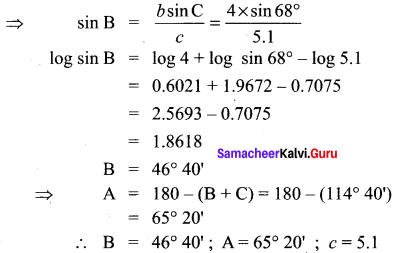# Samacheer Kalvi 11th Maths Solutions Chapter 3 Trigonometry Ex 3.10

## Tamilnadu Samacheer Kalvi 11th Maths Solutions Chapter 3 Trigonometry Ex 3.10

Question 1.
Determine whether the following measurements produce one triangle, two triangles or no triangle:
∠B = 88°, a = 23, b = 2. Solve if solution exists.
Solution:
We are given a = 23,
b = 2, and
∠B = 88°.
So we can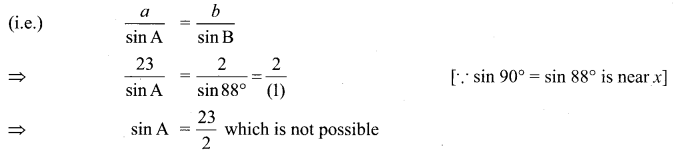Question 2.
If the sides of a ∆ABC are a = 4, b = 6 and c = 8, then show that 4 cos B + 3 cos C = 2.
Solution:
a = 4,
b = 6,
c = 8
To prove 4 cos B + 3 cos C = 2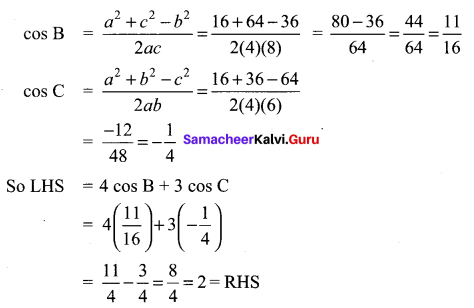Question 3.
In a ∆ABC, if a = $$\sqrt{3}$$ – 1, b = $$\sqrt{3}$$ + 1 and C = 60°, find the other side and other two angles.
Solution: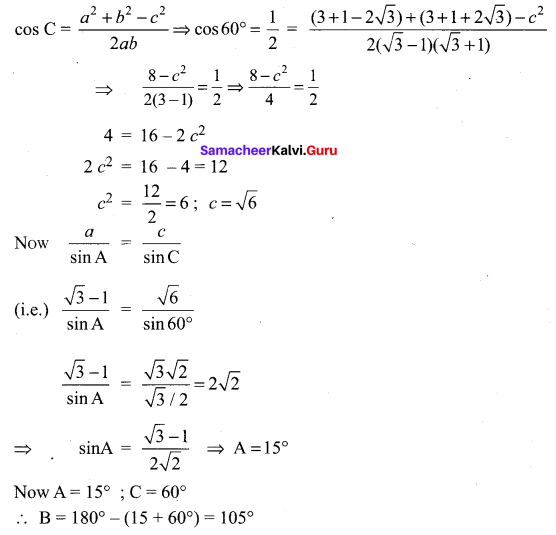Question 4.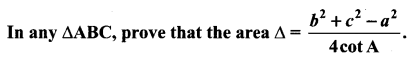Solution: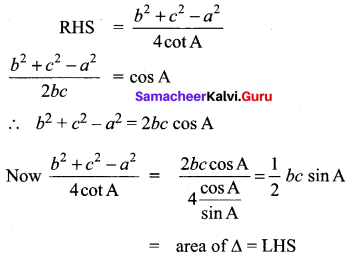Question 5.
In a ∆ABC, if a = 12 cm, b = 8 cm and C = 30°, then show that its area is 24 sq.cm.
Solution:
a = 12 cm,
b = 8 cm,
C = 30°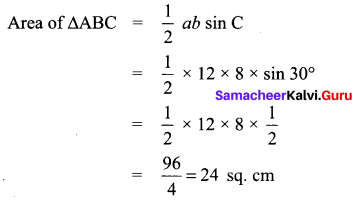Question 6.
In a ∆ABC, if a = 18 cm, b = 24 cm and c = 30 cm, then show that its area is 216 sq.cm.
Solution:
a = 18 cm,
b = 24 cm,
c = 30 cm
The sides form a right angled triangle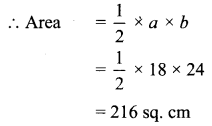Question 7.
Two soldiers A and B in two different underground bunkers on a straight road, spot an intruder at the top of a hill. The angle of elevation of the intruder from A and B to the ground level in the eastern direction are 30° and 45° respectively. If A and B stand 5 km apart, find the distance of the intruder from B.
Solution:
By using sine formula
$$\frac{x}{\sin 30^{\circ}}=\frac{5}{\sin 15^{\circ}}$$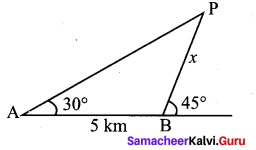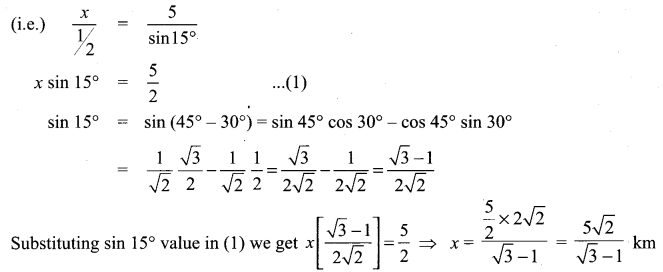Question 8.
A researcher wants to determine the width of a pond from east to west, which cannot be done by actual measurement. From a point P, he finds the distance to the eastern-most point of the pond to be 8 km, while the distance to the western most point from P to be 6 km. If the angle between the two lines of sight is 60°, find the width of the pond.
Solution:
p2 = W2 + E2 – 2WE cos P
P2 = 64 + 36 – 2 × 8 × 6 × Cos 60°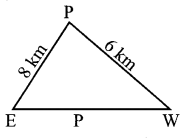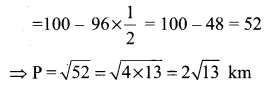Question 9.
Two Navy helicopters A and B are flying over the Bay of Bengal at same altitude from the sea level to search a missing boat. Pilots of both the helicopters sight the boat at the same time while they are apart 10 km from each other. If the distance of the boat from A is 6 km and if the line segment AB subtends 60° at the boat, find the distance of the boat from B.
Solution: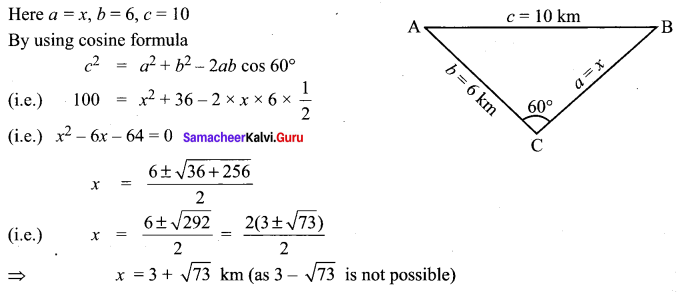Question 10.
A straight tunnel is to be made through a mountain. A surveyor observes the two extremities A and B of the tunnel to be built from a point P in front of the mountain. If AP = 3 km, BP = 5 km and ∠APB = 120°, then find the length of the tunnel to be built.
Solution:
p2 = a2 + b2 – 2ab cos P
p2 = 9 + 25 – 30 Cos 120°
p2 = 9 + 25 – 30 (-1/2) = 34 + 15 = 49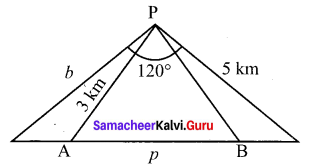⇒ p = $$\sqrt{49}$$ = 7 km

Question 11.
A farmer wants to purchase a triangular shaped land with sides 120 feet and 60 feet and the angle included between these two sides is 60°. If the land costs ? 500 per sq.ft, find the amount he needed to purchase the land. Also find the perimeter of the land.
Solution: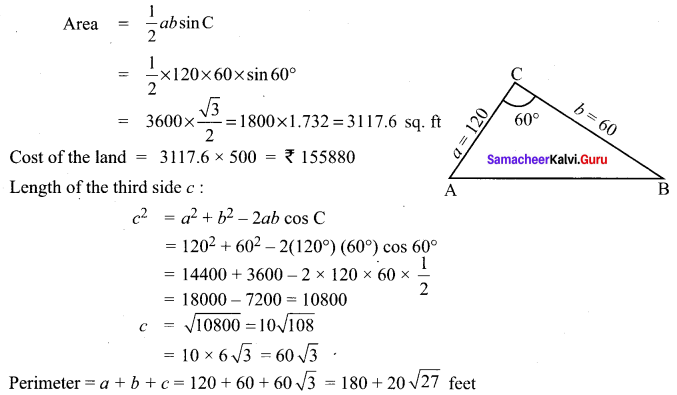Question 12.
A fighter jet has to hit a small target by flying a horizontal distance. When the target is sighted, the pilot measures the angle of depression to be 30°. If after 100 km, the target has an angle of depression of 60°, how far is the target from the fighter jet at that instant?
Solution: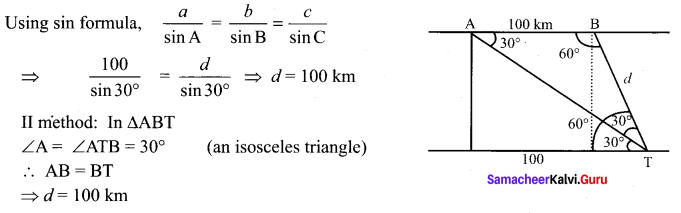Question 13.
A plane is 1 km from one landmark and 2 km from another. From the planes point of view the land between them subtends an angle of 60°. How far apart are the landmarks?
Solution: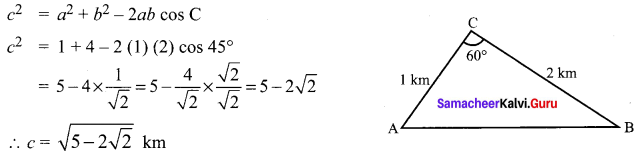Question 14.
A man starts his morning walk at a point A reaches two points B and C and finally back to A such that ∠A= 60° and ∠B = 45°, AC = 4 km in the ∆ABC. Find the total distance he covered during his morning walk.
Solution: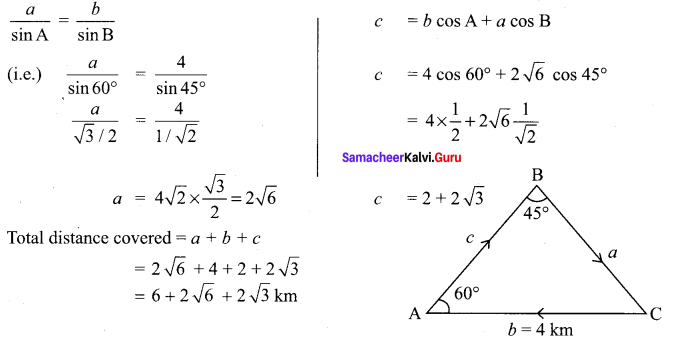Question 15.
Two vehicles leave the same place P at the same time moving along two different roads. One vehicle moves at an average speed of 60 km/hr and the other vehicle moves at an average speed of 80 km/hr. After half an hour the vehicle reach the destinations A and B. If AB subtends 60° at the initial point P, then find AB.
Solution: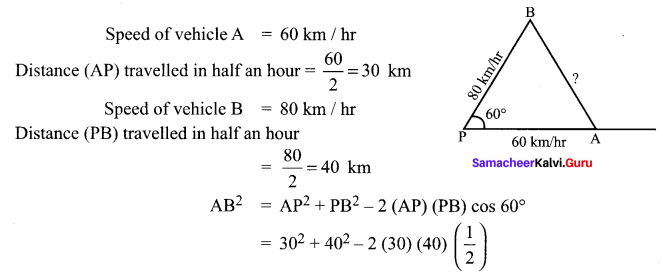= 900+ 1600 – 1200 = 1300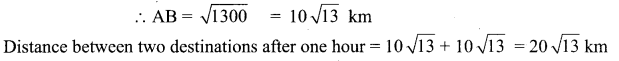Question 16.
Suppose that a satellite in space, an earth station and the centre of earth all lie in the same plane. Let r be the radius of earth and R be the distance from the centre of earth to the satellite. Let d be the distance from the earth station to the satellite. Let 30° be the angle of elevation from the earth station to the satellite. If the line segment connecting earth station and satellite subtends angle α at the centre of earth, then prove that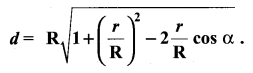Solution: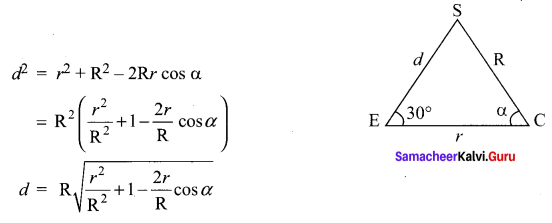### Samacheer Kalvi 11th Maths Solutions Chapter 3 Trigonometry Ex 3.10 Additional Questions Solved

Question 1.
Given a = 8, b = 9, c = 10, find all the angles.
Solution: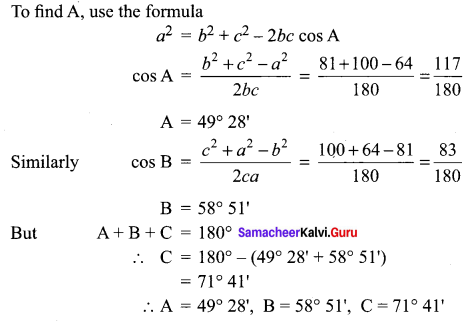Question 2.
Given a = 31, b = 42, c = 57, find all the angles.
Solution:
Since the sides are larger quantities, use half angles formulae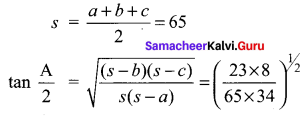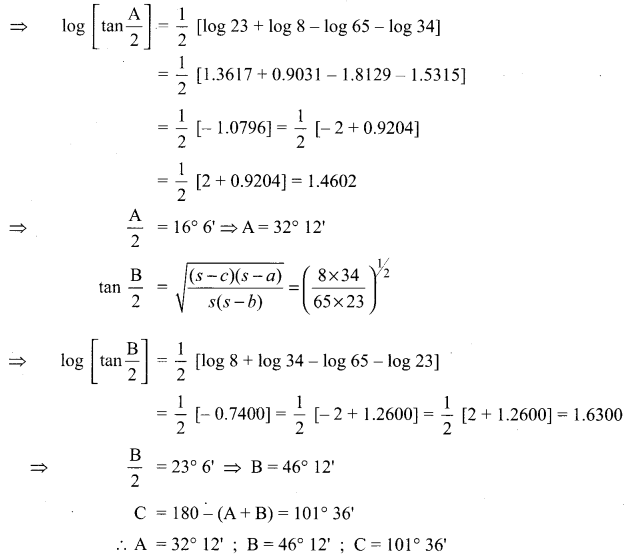Question 3.
In a triangle ABC, A = 35° 17′ ; C = 45° 13′ ; b = 42.1 Solve the triangle
Solution:
The unknown parts are B, a, c,
B = 180 – (A + C) = 180 – (35° 17′ + 45° 13′)
= 99° 30′
To find sides, use sine formula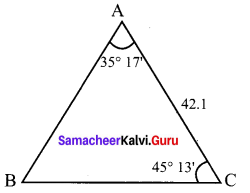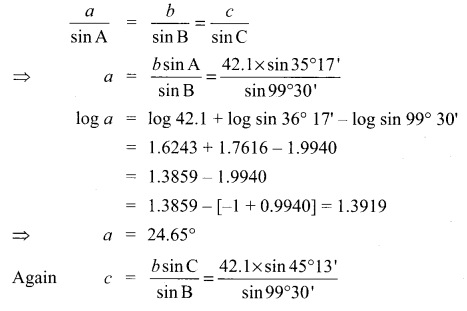log c = log 42.1 + log sin 45° 31 – log sin 99° 30′
= 1.6243 + 1.8511 – 1.9940
= 1.4754 – 1.9940
= 1.4754 – [-1 + 0.9940] = 1.4814
⇒ c = 30.3°
Thus B = 99° 30′ ; a = 24.65° ; c = 30.3°Question 4.
Solve the triangle ABC if a = 5, b = 4 and C = 68°.
Solution:
To find c, use c2 = a2 + b2 – 2ab cos C
c2 = 25 + 16 – 2 × 5 × 4 cos 68°
= 41 – 40 × 0.3746 = 26.016
c = 5.1
To find the other two angles, use sine formula.